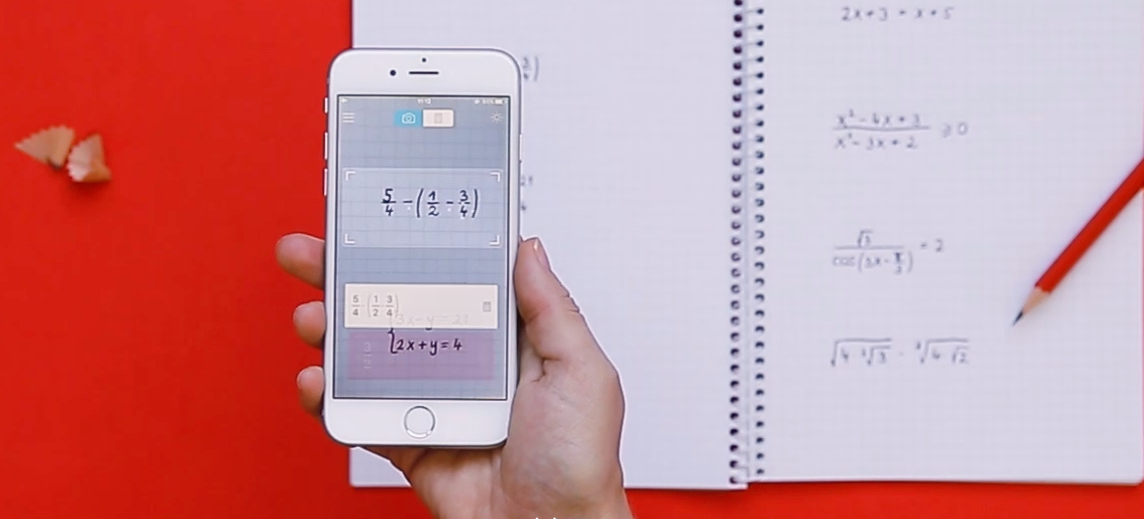# How to solve math problems with iPhone, iPad or Android: fractions, roots, trigonometry, logarithms, systems of equations and other calculations

Some of us seem to be sorry we did not have one in our time iPhone or iPad able to do us all the themes of mathematics and us solves the problems in the collections, no matter how complicated they may be.

Not so long ago, the application Calculator a iPhone or Android was the only one that could help us with the calculations simpl. Even if in "landscape" mode it goes on scientific calculator.

As technology evolved and the imagination of developers developed, "Calculator" applications began to appear. able to scan the sheet of a math book si deal and most complicated calculations. everything through it simplease scan.
Basically, if you have written in a booklet on a sheet or in a collection, an exercise with fractions, equations with square inequalities. absolute, linearwith exponential functions or logarithmic and other math crazes like this, all you have to do is install the application Photomath, open it and scan the port on the page where the exercise you want to resolve is written.Of course, the application also works if you want to scan an exercise from the PC, laptop or iPad monitor.

What kind of expressions / exercises can be solved with Photomath

Photomath has published a PDF file with a list containing example exercises, which can be solved by simplease scan with the application. Find the file here. fractions, decimal numbers, algebraic expressions, roots, equations (linear inequalities, pathetic inequalities, absolute inequalities), equation systems, logarithms, trigonometry, exponential functions si logarithmic, derived and integral.

##### Photomath - Camera Calculator
• Accuracy Solutions Exercises
• Graphical User Interface
• Smartphone Compatibility
4.7

Photomath - Camera Calculator

Solve math exercises by simscan the exercise / expression from the page of the math notebook, from a sheet or from a collection. Fractions, decimal numbers, algebraic expressions, roots, equations (linear inequalities, quadratic inequalities, absolute inequalities), systems of equations, logarithms, trigonometry, exponential and logarithmic functions, derivatives and integrals.

##### User Review